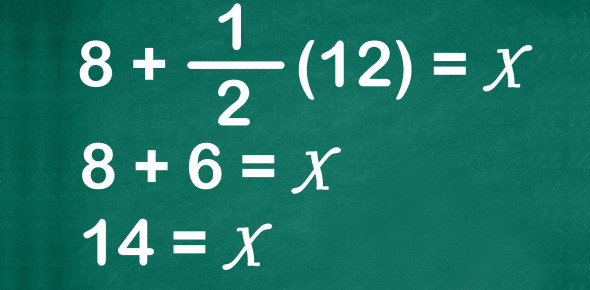# Quiz: Can You Solve These Maths Problems?

8 Questions | Attempts: 1581945SettingsCan you solve these maths problems? Math is an awesome subject, and not everyone is a fan of a math test or class. The quiz below is designed to help you get familiar with some fundamental math problems.

• 1.
What is the square root of 49?
• A.

16

• B.

45

• C.

12

• D.

7

• E.

10

• 2.
If I have a cirle with a radius of 50yds, what is the diameter?
• A.

25yds

• B.

314yds

• C.

100yds

• 3.
What happens to the area of a rectangle when you double the legth of both sides?
• A.

Area is 2x

• B.

Area is 4x

• C.

Area is 6x

• 4.
List one dimention of a rectangular prism with the wolume of 36ft3?
• A.

2x4x4.5

• B.

2x8x5

• C.

9x6x3

• D.

7x10x4

• E.

67x4x90

• 5.
If you are selling 15 c-ds to 3 costomers how many c-d does each customer have?
• A.

4

• B.

8

• C.

13

• D.

2

• E.

5

• 6.
Pythagoras theorem states:
• A.

The product of the square of lengths hypotenuse, base and perpendicular is equal to the sum of the angles.

• B.

The square of the hypotenuse is equal to the sum of the base and the perpendicular in a right angled triangle.

• C.

The square of the base is equal to the sum of the squares of the other two sides.

• D.

The square of the hypotenuse (the side opposite the right angle) is equal to the sum of the squares of the other two sides.

• 7.
Sinθ/cosθ =
• A.

1

• B.

Cotθ

• C.

Tanθ

• D.

Secθ

• 8.
A grocer sells 100 kg of jaggery partly at 10% profit and partly at 20% profit, gaining 12% on his outlay. How much does he sell at 10% profit ?
• A.

80 Kg

• B.

88 Kg

• C.

75 Kg

• D.

None of the above

## Related TopicsBack to top
×

Wait!
Here's an interesting quiz for you.